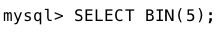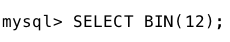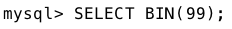# MySQL | BIN() Function

• Last Updated : 03 Mar, 2018

The BIN() function in MySQL is used to convert a decimal number to its binary equivalent.The BIN() function is equivalent to the CONV() function written in the format CONV(number,10,2). In this format CONV() function converts the number ‘number’ from base 10(decimal) to base 2(binary).

Syntax :

Attention reader! Don’t stop learning now. Learn SQL for interviews using SQL Course  by GeeksforGeeks.

```BIN( NUM )
```

Parameters Used:
NUM:It is the decimal number whose binary equivalent you want to find.

Return Value:
The BIN() function returns a string value, the binary equivalent of the decimal number passed as argument. It returns NULL if the number is NULL.

Examples:

1. Converting 5 to its binary equivalent.
Syntax:Output:
`'101'`

2. Converting 12 to its binary equivalent.
Syntax:Output:
`'1100'`

3. Converting 97 to its binary equivalent.
Syntax:Output:
`'1100001'`

My Personal Notes arrow_drop_up